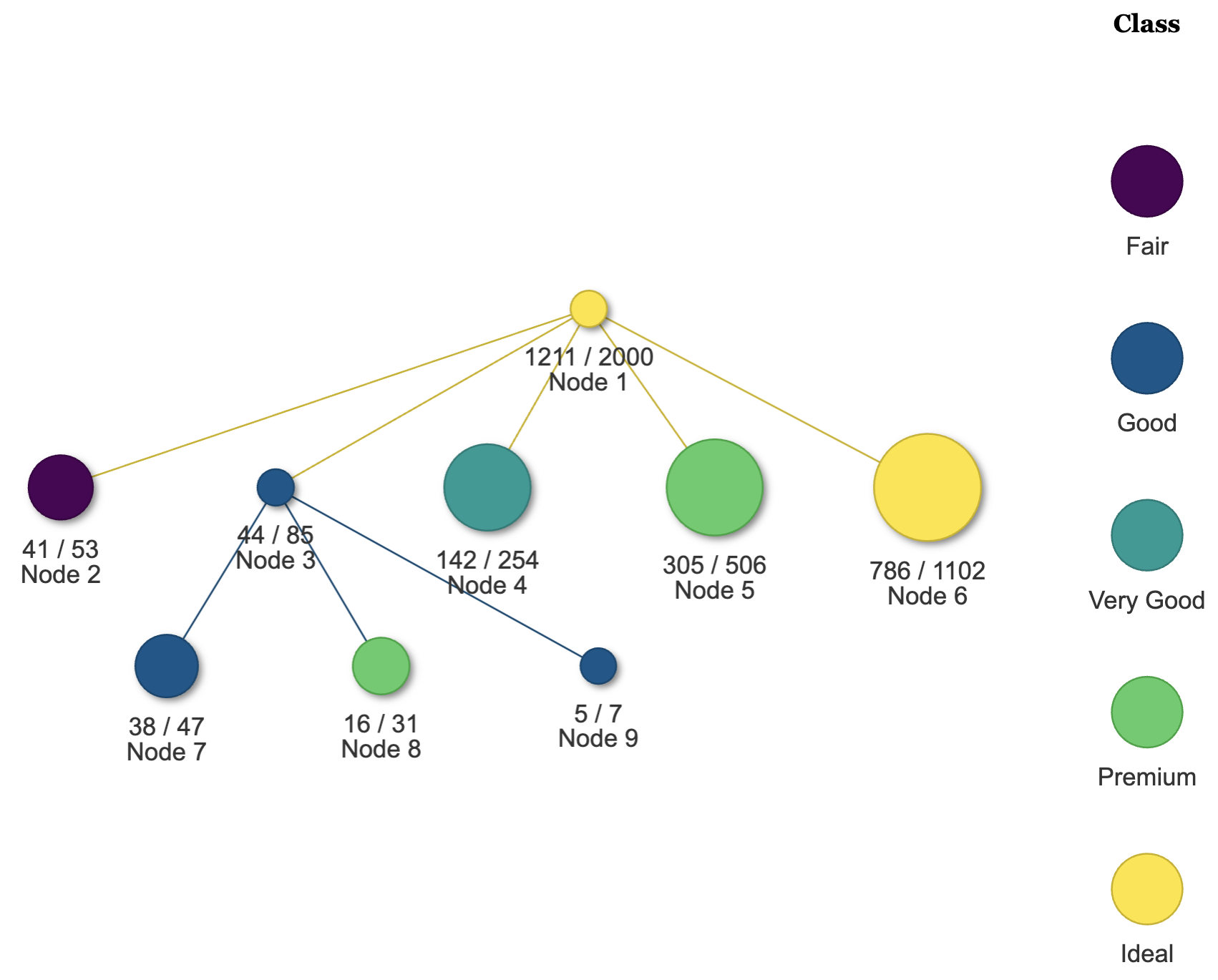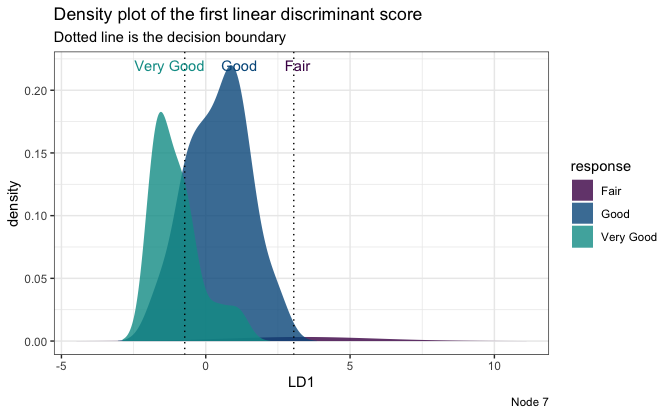LDATree is an R modeling package for fitting classification trees. If you are unfamiliar with classification trees, here is a tutorial about the traditional CART and its R implementation rpart.

## Overview

Compared to other similar trees, LDATree sets itself apart in the following ways:

• It applies the idea of LDA (Linear Discriminant Analysis) when selecting variables, finding splits, and fitting models in terminal nodes.

• It addresses certain limitations of the R implementation of LDA (MASS::lda), such as handling missing values, dealing with more features than samples, and constant values within groups.

• Re-implement LDA using the Generalized Singular Value Decomposition (GSVD), LDATree offers quick response, particularly with large datasets.

• The package also includes several visualization tools to provide deeper insights into the data.

## Installation

install.packages("LDATree")

## Usage

To build an LDATree:

library(LDATree)

fit <- Treee(Species~., data = iris)

To plot the LDATree:

# View the overall tree.
plot(fit)# Three types of individual plots
# 1. Scatter plot on first two LD scores
plot(fit, data = iris, node = 1)# 2. Density plot on the first LD score
plot(fit, data = iris, node = 3)
#> Warning: Groups with fewer than two data points have been dropped.
#> Warning in max(ids, na.rm = TRUE): no non-missing arguments to max; returning
#> -Inf# 3. A message
plot(fit, data = iris, node = 5)
#>  "Every observation in this node is predicted to be virginica"

To make predictions:

# Prediction only.
predictions <- predict(fit, iris)
#>  "setosa" "setosa" "setosa" "setosa" "setosa" "setosa"
# A more informative prediction
predictions <- predict(fit, iris, type = "all")
#> 6   setosa   13      0          0         0